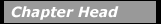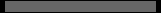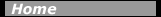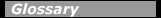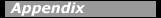## Nuclear ReactionsNuclear reactions and nuclear scattering are used to measure the properties of nuclei. Reactions that exchange energy or nucleons can be used to measure the energies of binding and excitation, quantum numbers of energy levels, and transition rates between levels. A particle accelerator (see Chapter 11) produces a beam of high-velocity charged particles (electrons, protons, alphas, or "heavy ions"), which then strikes a target nucleus. Nuclear reactions can also be produced in nature by high-velocity particles from cosmic rays, for instance in the upper atmosphere or in space. Beams of neutrons can be obtained from nuclear reactors or as secondary products when a charged-particle beam knocks out weakly-bound neutrons from a target nucleus. Nuclear reactions can also be produced by beams of photons, mesons, muons, and neutrinos.

In order for a nuclear reaction to occur, the nucleons in the incident particle, or projectile, must interact with the nucleons in the target. Thus the energy must be high enough to overcome the natural electromagnetic repulsion between the protons. This energy "barrier" is called the Coulomb barrier. If the energy is below the barrier, the nuclei will bounce off each other. Early experiments by Rutherford used low-energy alpha particles from naturally radioactive material to bounce off target atoms and measure the size of the target nuclei.

When a collision occurs between the incident particle and a target nucleus, either the beam particle scatters elastically leaving the target nucleus in its ground state or the target nucleus is internally excited and subsequently decays by emitting radiation or nucleons. A nuclear reaction is described by identifying the incident particle, target nucleus, and reaction products. For example, when a neutron strikes a nitrogen nucleus, 14N, to produce a proton, 1H, and an isotope of carbon, 14C, the reaction is written as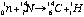Sometimes the reaction is abbreviated as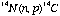. A number of conservation conditions apply to any reaction equation:

• The mass number A and the charge Z must balance on each side of the reaction arrow. Thus in the example the sum of superscripts 1 + 14 on the left equals the 14 + 1 on the right to balance the A’s. The 0 + 7 subscripts on the left equals the 6 + 1 on the right to balance the Z’s.
• The total energy before the reaction must equal the total energy after the reaction. The total energy includes the particle kinetic energies plus the energy equivalent of the particle rest masses, E = mc 2.
• Linear momenta before and after the reaction must be equal. For two-particle final states this means that a measurement of one particle’s momentum determines the other particle’s momentum.
• Quantum rules govern the balancing of the angular momentum, parity, and isospin of the nuclear levels.

A specific reaction is studied by measuring the angles and kinetic energies of the reaction products (the kinematic variables). Particle and radiation detectors designed for the expected charge and energy of each product are arranged around the target. (See chapter 12 for a discussion of detector types.)

The most important quantity of interest for a specific set of kinematic variables is the reaction cross section. The cross section is a measure of the probability for a particular reaction to occur. This quantity, s, which has the dimension of area, is measured by the experimental ratio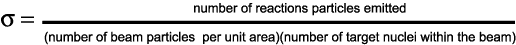The cross section can also be calculated from a mathematical model of the nucleus by applying the rules of quantum mechanics. Comparing the measured and calculated values of the cross sections for many reactions validates the assumptions of the nuclear model.

Table 7-1 shows some of the many types of nuclear reactions and what they teach us about nuclei and nuclear energy.

Table 7-1. Nuclear Reaction Types

 ReactionWhat is learnedNucleon- nucleon scattering Fundamental nuclear force Elastic scattering of nuclei Nuclear size and interaction potential Inelastic scattering to excited states Energy level location and quantum numbers Inelastic scattering to the continuum Giant resonances (vibrational modes) Transfer and knockout reactions Details of the Shell Model Fusion reactions Astrophysical processes Fission reactions Properties of Liquid-drop Model Compound nucleus formation Statistical properties of the nucleus Multifragmentation Phases of nuclear matter, Collective Model Pion reactions Investigation of the nuclear "glue" Electron scattering Quark structure of nuclei

The elastic scattering cross sections of protons and neutrons on a proton target give the essential data to reconstruct the nucleon-nucleon. A complete theory of nuclear structure and dynamics must start with this elemental interaction.

The systematics of nuclear sizes, shapes, binding energies, and other nuclear properties are the data that nuclear models are challenged to explain. The Shell Model mentioned in Chapters 2 and 6 has combined the large body of nuclear data into a coherent theory of nuclear structure. Most of this data was the result of elastic and inelastic scattering of electrons, protons, and neutrons from nuclei found in the chart of the nuclides.

At high enough excitation energies, a nucleus can undergo a series of normal modes of collective oscillations called giant resonances. The nucleus rings like a bell at distinct frequencies with all the nucleons participating and sharing the excitation energy.

Fusion reactions are the combining of two nuclei to form a more massive nucleus. Many fusion reactions release large amounts of energy. An example is the combining of two isotopes of hydrogen (tritium and deuterium) to form helium and a neutron plus a large amount of kinetic energy in the reaction products:

3H + 2H Æ 4He + n + 17.6 MeV.

This reaction as a potential electric power source is discussed in Chapter 14. Another example of fusion is the reaction set that powers the Sun and other low-mass stars:

1H + 1H Æ 2H + e+ + n e,

2H + 1H Æ 3He + g,

3He + 3He Æ 4He +1H + 1H.

The net energy output from this chain is 26.7 MeV for each helium-4 nucleus formed.

Neutron-induced fission of massive nuclei into two lower-mass nuclei plus neutrons is also an energy source for power generation. This topic is discussed in Chapter 14.

Compound nucleus formation is a reaction in which two nuclei combine into a single excited nucleus; the excited nucleus lives for a relatively long time and "forgets" how it was formed. The decay from this state of excitation is by "evaporation" of nucleons from the heated liquid drop of the compound nucleus, by gamma decay, or by fission of the compound nucleus. The statistical nature of this process teaches us about the average properties of excited states of complex nuclei.

Multifragmentation reactions, in which high-energy nuclei collide with other nuclei, are a method of creating nuclear matter in unusual conditions of density and excitation energy. These states may be in a different phase from normal nuclei and be characteristic of the matter in the early universe.

The fundamental force between nucleons in nuclei is dominated by the exchange of p mesons (pions). When these particles are created in high-energy proton reactions, they can be used to bombard nuclear targets. When the pion interacts with a nucleus, it forms a resonance with one of the bound nucleons. The resonance is shifted and broadened compared to the reaction on a free nucleon. These changes reflect the influence of the neighboring nucleons.

When nucleons are flung at one another, they can mesh briefly. During the time they are one nucleus, the quarks in the nucleons can interact with one another as if they were free particles. As with Rutherford scattering, an investigation of the angle that a particle is scattered gives information about the conditions inside the nucleons.

Nuclear reactions and their interpretation are the main activity of most nuclear scientists. The continuing development of accelerators and detectors (see Chapters 11 and 12) permit the refinement of nuclear data and models to benefit basic science and nuclear applications.

#### Chapter Contents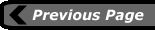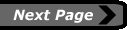•   last updated: January 25, 2001 webmaster